# Text : Multiline data entry widget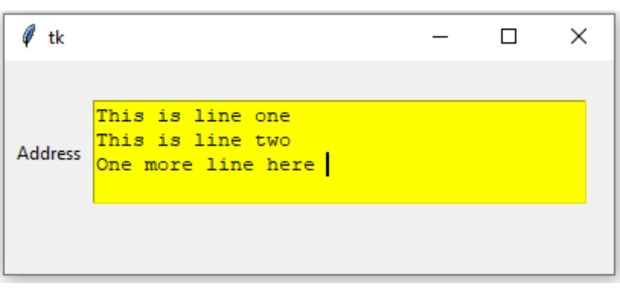We will keep one Label along with a text box.

Tkinter Text style & to read input & display text on click event of button by get() & set() methods

User input for multiline of text. ( for single line of text use Entry)
``t1=tk.Text(parent_window,options)``
`parent_window` : Declared Parent window
`options` : Various options can be added, list is given below.
``````import tkinter as tk
my_w = tk.Tk() # Parent window
my_w.geometry("400x180") # Width height of parent window

t1 = tk.Text(my_w, width=40,bg='yellow' , height=4) # Text
t1.grid(row=1,column=2)  # placed on grid

my_w.mainloop()``````
To manage layout read more on grid()

## Total number of chars inside the Text widget

Reading data of text box starting from first char till end
``t1.get("1.0",END)``
"1.0" mean Line 1 , first char. Similarly END is the string end including the line break.
To remove line break at the end we have to modify like this.
``t1.get("1.0",'end-1c')``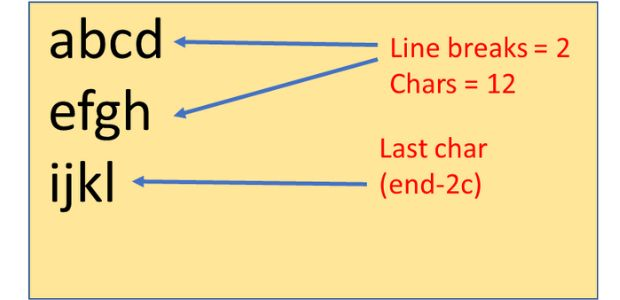For a multi-line text above code will remove line break at the last line only. To calculate the total number of chars the user has entered inside the text widget, we have to count the number of line breaks ( excluding the last one ).
len() : Return the number of elements present in an iterable
count() : Counts the number of matching string present.
``````my_str=t1.get("1.0",'end-1c') # excluding the last line break char.
breaks=my_str.count('\n')  # Number of line breaks ( except last one )
char_numbers=len(my_str)-breaks # total chars excluding line breaks``````

## Deleting last char inside Text widget

``t1.delete('end-2c')``

Adding data to a text box my_str1 is the string variable to insert.
``t2.insert(tk.END, my_str1)``
Above codes are important to develop applications.

Note that the widely used option textvariable is not available with Text widget

There are many options we can add to text box, the list is available below.

Now let us add the click event of the button linked to text box. We will enter data in our text box , on click of the button the entered data will be displayed at another Label.
``````import tkinter as tk
from tkinter import BOTH, END, LEFT
my_w = tk.Tk()
my_w.geometry("500x300")

def my_upd():
my_str.set(t1.get("1.0",END))  # read the text box data and update the label

my_str = tk.StringVar()

l1.grid(row=1,column=1)

t1 = tk.Text(my_w,  height=1, width=20,bg='yellow')# added one text box
#t1=Entry(my_w, width=10)
t1.grid(row=1,column=2)

b1 = tk.Button(my_w, text='Update', width=10,bg='red',command=lambda: my_upd()) # button added
b1.grid(row=1,column=3)

l2 = tk.Label(my_w,  textvariable=my_str, width=20 ) # added one Label
l2.grid(row=1,column=4)
my_str.set(" I will update")

my_w.mainloop()``````
Let us update one text box by using data from another text box.
``````import tkinter as tk
from tkinter import BOTH, END, LEFT
my_w = tk.Tk()
my_w.geometry("500x400")

def my_upd():
my_str1=t1.get("1.0",END)  # read from one text box t1
t2.insert(tk.END, my_str1) # Add to another text box t2

my_str = tk.StringVar()

l1.grid(row=1,column=1)

t1 = tk.Text(my_w,  height=1, width=20,bg='yellow')# added one text box
#t1=Entry(my_w, width=10)
t1.grid(row=1,column=2)

b1 = tk.Button(my_w, text='Update', width=10,bg='red',command=lambda: my_upd()) # button added
b1.grid(row=1,column=3)

t2 = tk.Text(my_w, height=1, width=15, bg='#00f000' ) # added one textbox to read
t2.grid(row=1,column=4)

my_w.mainloop()``````

## Delete ( or empty ) text box

We can remove data from first text box after updating the second text box. In above code you can two more lines inside the function.
``````def my_upd():
my_str1=t1.get("1.0",END)  # read from one text box t1
t2.insert(tk.END, my_str1) # Add to another text box t2
t1.delete('1.0',END)       # Delete from position 0 till end
t1.update()                # update the delete``````

## Remove all inputs from all text widgets

Irrespective of number of Text boxes we used in our application, we can remove all user entered data by using winfo_children() which returns all the widget classes of the Tkinter window. Here is the code
``````for widget in my_w.winfo_children():
if isinstance(widget, tk.Text):  # If this is an Entry widget
widget.delete(0,'end') # Delete all entries ``````

## Focus text box

To keep the ( blinking ) cursor inside text box
``t1.focus() ``

## Options can be used in a textboxbg Background colour of the text box``t1 = tk.Text(my_w, width=8, height=1, bg='yellow')`` We can update the background colour by using configure() We have two radio buttons , on select of radio buttons we can change the background colour of the text box. t1.configure(bg=r1_v.get()) Read more on Radio buttons Full code is here ``````import tkinter as tk my_w = tk.Tk() my_w.geometry("200x200") l1 = tk.Label(my_w, text='Your Name' ) # added one Label l1.grid(row=1,column=1) t1 = tk.Text(my_w, width=8, height=1) t1.grid(row=1,column=2) ###### Radio buttons ####### def my_upd(): t1.configure(bg=r1_v.get()) # update the colour r1_v = tk.StringVar() # We used string variable here r1_v.set('Yellow') # Can assign value Appear or Failed r1 = tk.Radiobutton(my_w, text='Yellow', variable=r1_v, value='Yellow',command=my_upd) r1.grid(row=2,column=1) r2 = tk.Radiobutton(my_w, text='Red', variable=r1_v, value='Red',command=my_upd) r2.grid(row=2,column=2) my_w.mainloop()``````
 bd Border around the Text. Default width is 2``t1 = tk.Text(my_w, width=8, height=1, bd=6)``
 cursor cursor that will appear once the mouse is over the text box ``t1 = tk.Text(my_w, width=8, height=1,cursor='circle')`` You can get a list of cursor shapes in our Tkinter button page
 exportselection Selection is to be exported or not. The default is usually for widgets to export selection. ``````t1 = tk.Text(my_w, width=20, height=1, exportselection=1)``````
 font Managing font size, family, type etc ``t1 = tk.Text(my_w, width=20, height=1,font=18)`` font size with family ``````my_font=('times', 8, 'bold') t1 = tk.Text(my_w, width=20, height=1,font=my_font)`````` Zoom in & Zoom out of Text by using font size Managing font using Menu bar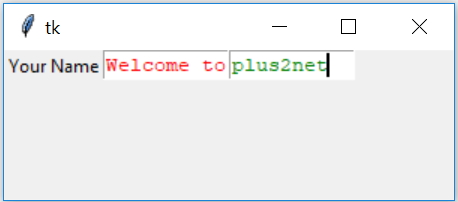fg Colour used for fg ``````l1 = tk.Label(my_w, text='Your Name' ) # added one Label l1.grid(row=1,column=1) t1 = tk.Text(my_w, width=10, height=1,fg='red') t1.grid(row=1,column=2) t2 = tk.Text(my_w, width=10, height=1,fg='green') t2.grid(row=1,column=3) ``````
 height Height in number of lines ``t1 = tk.Text(my_w, width=10, height=2)``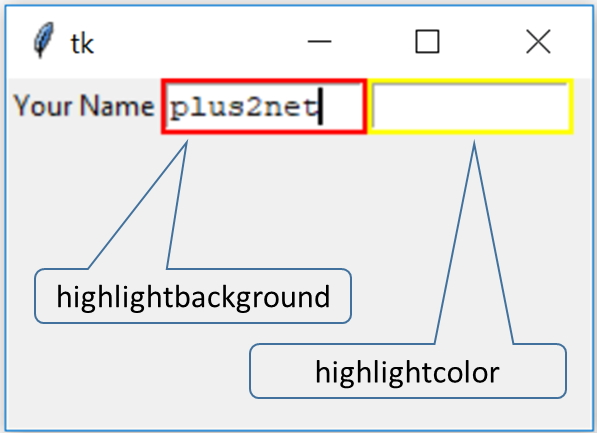highlightbackground
 focus highlight color ( the rectangle around the text box ) when text box is not in focus. ( Note keep highlightthickness = 2 to get a visible output of the rectangle around the text box ) ``t1 = tk.Text(my_w, width=10,height=1,highlightbackground='red')``
highlightcolor
 focus highlight ( the rectangle around the text box ) color when text box is in focus ``t1 = tk.Text(my_w, width=10,height=1,highlightcolor='red')``

highlightthickness
 The width of the focus heighlight. ( the rectangle around the text box ) Default value is 1. ``t1 = tk.Text(my_w, width=10,height=1,highlightthickness=2)``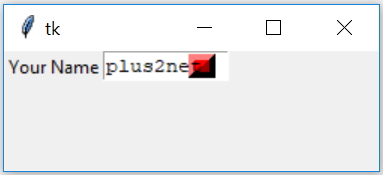insertbackground
 Blinking Cursor background colour. Here we kept `insertwidth=15` to make the cursor thick enough to make it visible. We have changed `insertborderwidth=10` to make the 3 D effect visible. ``````t1 = tk.Text(my_w,width=10,height=1, insertbackground='red',insertwidth=15)``````

insertborderwidth
 Widht of the border of Cursor to give 3 D Effect. ``````t1 = tk.Text(my_w,width=10,height=1, insertborderwidth=8, insertwidth=18)``````
insertofftime
 The off time in milliseconds for the blinking cursor. For 0 value it will not blink. ``````t1 = tk.Text(my_w,width=10,height=1, insertofftime=1000,insertontime=1000)``````
insertontime
 The on time in milliseconds for the blinking cursor. ``````t1 = tk.Text(my_w,width=10,height=1, insertofftime=1000,insertontime=1000)``````
insertwidth
 Width of the blinking cursor ``t1 = tk.Text(my_w, width=10,height=1,insertwidth=15)``
 justify Text justification . Values can be LEFT RIGHT or CENTER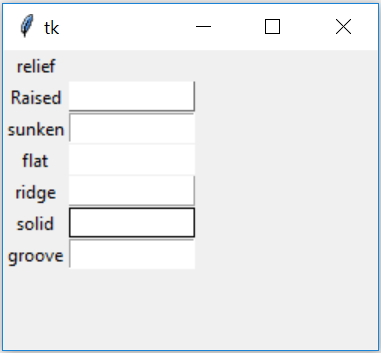relief The text box 3 D effect style. It can take these values raised , sunken ,flat, ridge, solid & groove ``````import tkinter as tk my_w = tk.Tk() my_w.geometry("250x200") l1 = tk.Label(my_w, text='relief' ) # added one Label l1.grid(row=1,column=1) l1 = tk.Label(my_w, text='Raised' ) # added one Label l1.grid(row=2,column=1) t1 = tk.Text(my_w, width=10,height=1,relief='raised') t1.grid(row=2,column=2) l1 = tk.Label(my_w, text='sunken' ) # added one Label l1.grid(row=3,column=1) t1 = tk.Text(my_w, width=10,height=1,relief='sunken') t1.grid(row=3,column=2) l1 = tk.Label(my_w, text='flat' ) # added one Label l1.grid(row=4,column=1) t1 = tk.Text(my_w, width=10,height=1,relief='flat') t1.grid(row=4,column=2) l1 = tk.Label(my_w, text='ridge' ) # added one Label l1.grid(row=5,column=1) t1 = tk.Text(my_w, width=10,height=1,relief='ridge') t1.grid(row=5,column=2) l1 = tk.Label(my_w, text='solid' ) # added one Label l1.grid(row=6,column=1) t1 = tk.Text(my_w, width=10,height=1,relief='solid') t1.grid(row=6,column=2) l1 = tk.Label(my_w, text='groove' ) # added one Label l1.grid(row=7,column=1) t1 = tk.Text(my_w, width=10,height=1,relief='groove') t1.grid(row=7,column=2) my_w.mainloop()``````
 selectbackground The background colour of the selected text inside the textbox. ``````t1 = tk.Text(my_w, width=10,height=1, selectbackground='red')`````` selectborderwidth The width of the boarder around selected text selectforeground The colour of the font of selected text ``````t1 = tk.Text(my_w, width=10,height=1, selectforeground='yellow')`````` width Width in number of chars``t1 = tk.Text(my_w, height=1, width=20)`` xscrollcommand Add scroll bar to text box wrap values can be char, none, word How to use Checkbutton to wrap text in Entry widget

## Methods

Delete chars from start position to end position
``l2.delete(1.4,1.7) # from 4th till 7th position of line one``
``l2.delete(1.0,END) # from starting till end``

## get()

``````print(l2.get(1.4,1.7)) # print from 4th till 7th position of line one
print(l2.get(1.0,END))  # Print from starting till end ``````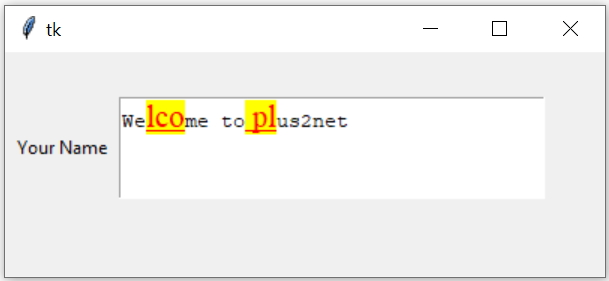``````import tkinter as tk
from tkinter import BOTH, END, LEFT
my_w = tk.Tk()
my_w.geometry("400x300")
l1.grid(row=1,column=1)

t1 = tk.Text(my_w,width=35,height=4) #text box

font1=('Times',16,'underline')
t1.insert(tk.END, "Welcome to plus2net")

t1.tag_add("my_hg", "1.2", "1.13") # tag name my_hg is created
t1.tag_config("my_hg",background="yellow",foreground="red",font=font1)
t1.tag_remove('my_hg','1.5','1.10') # tag is removed from part

my_w.mainloop()``````

## Using buttons to trigger events using tags

We can destroy the tag by using tag_delete(). Similarly we can use tag_remove() and tag_config() by using button clicks.
``````#t1.tag_delete('my_hg')
b1=tk.Button(my_w,text='Remove tag',command=lambda:t1.tag_remove('my_hg','1.0','1.7'))

b2=tk.Button(my_w,text='Green tag',command=lambda:t1.tag_config('my_hg',background="green",foreground="red",font=font1))

## Selecting all data from Text box

``````def select_all(): # to select all text inside Text box
e1.tag_add("sel", "1.0","end") # all text selected
e1.tag_config("sel",background="green",foreground="red")``````

1. Exercise on Text
2. Ask user to enter Name, marks ( in three subjects Physics, Chemistry, Math ) and attendance of a student. On Update , the data will be stored in a Dictionary and finally in a CSV file. ( Break it in parts, First display the data using print(), then create Dictionary and then save as CSV file. )
3. Create one user data entry box in the window and on click of the Save button the text entered in the entry box will be saved into one text file using the filedialog.
4. Save the above user entered data to one PDF file.

Subscribe to our YouTube Channel here

## Subscribe

* indicates required
Subscribe to plus2netplus2net.com